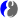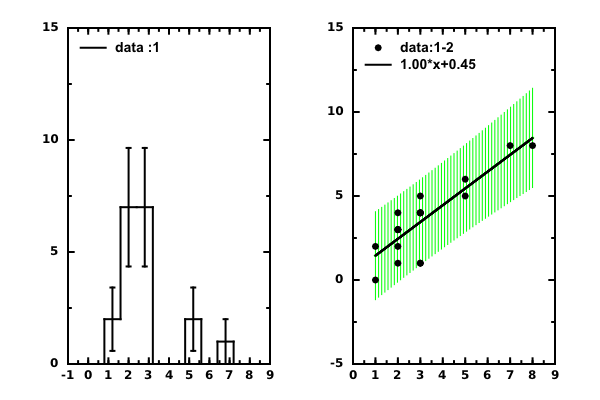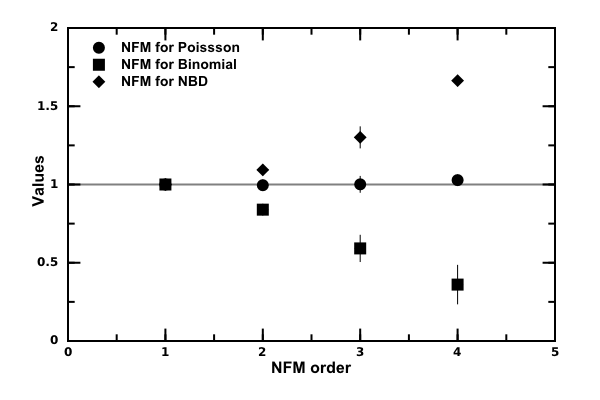# DMelt:Statistics/1 General Topics

Limitted access. First login to DataMelt if you are a full DataMelt member. Then login to HandWiki as a user.

# Statistics of 1D arrays

The packagejhplot.stat can be used for descriptive analysis of random distributions in 1D. Similarly,cern.jet.stat.Descriptive package contains descriptive methods to calculate many statistical characteristics.

Consider also several other packages:

But before using such packages, check again the data containers such asjhplot.P1D orjhplot.H1D . They already have many useful methods to access statistical information on data.

Some statistical questions have been already described before. Below we will consider several advances topics.

# Moments of a distribution

DataMelt can be used to determine statistical characteristics of an arbitrary frequency distribution. Moments of this distribution can be calculated up to the 6th order. Read more aboutMoment (mathematics).

from jhplot  import *
from jhplot.math.StatisticSample import *
a=randomLogNormal(1000,0,10) # generate random 1000 numbers between 0 and 10 using a LogNormal distribution
p0=P0D(a)                    # convert it to an array
print p0.getStatString()     # print detailed characteristics

To show this output, click expand

Size: 1000
Sum: 2.0795326321690155E11
SumOfSquares: 1.722072831288292E22
Min: 4.3681673233597326E-14
Max: 1.187289072883721E11
Mean: 2.0795326321690154E8
RMS: 4.1497865382309628E9
Variance: 1.7194678431631995E19
Standard deviation: 4.14664664899627E9
Standard error: 1.3112848062732975E8
Geometric mean: 0.7193930848008395
Product: 9.252494313364321E-144
Harmonic mean: 2.2976022239249118E-11
Sum of inversions: 4.352363475222163E13
Skew: 25.65476598759878
Kurtosis: 694.7699433878664
Sum of powers(3): 1.839916709064571E33
Sum of powers(4): 2.0782654881247146E44
Sum of powers(5): 2.4093597349729484E55
Sum of powers(6): 2.8286717081193334E66
Moment(0,0): 1.0
Moment(1,0): 2.0795326321690154E8
Moment(2,0): 1.722072831288292E19
Moment(3,0): 1.839916709064571E30
Moment(4,0): 2.0782654881247147E41
Moment(5,0): 2.409359734972948E52
Moment(6,0): 2.8286717081193336E63
Moment(0,mean()): 1.0
Moment(1,mean()): 4.931390285491944E-7
Moment(2,mean()): 1.7177483753200437E19
Moment(3,mean()): 1.8291913748162454E30
Moment(4,mean()): 2.0630054468429083E41
Moment(5,mean()): 2.3878300421487077E52
Moment(6,mean()): 2.798744135044988E63
25%, 50%, 75% Quantiles: 0.0012310644573427145, 0.9530465118707188, 535.0653267374155
quantileInverse(median): 0.5005
Distinct elements & frequencies not printed (too many).


Let us continue with this example and now we would like to return all statistical characteristics of the sample as a dictionary. We can do this by appending the following lines that 1) create a dictionary "stat" with key/value pairs; 2) retrieve a variance of the sample using the key Variance.

stat=p0.getStat()
print "Variance=",stat["variance"]

which will print "Variance= 757.3". If not sure about the names of the keys, simply print the dictionary as "print stat".

One can create histograms that catch the most basic characteristics of data. This is especially important if there is no particular reasons to deal with complete data arrays. We can easily do this with above Fibonacci sequence as:

h=p0.getH1D(10, 0, 100)
print h.getStat()

The code converts the array into a histogram with 10 equidistant bins in the range 0-100, and then it prints the map with statistical characteristics.

You can also visualize the random numbers in the form of a histogram as shown in this detailed example above. We create random numbers, convert them to histograms and plot them.

# Statistics with 2D arrays

You can get detailed statistics on 2D data represented byjhplot.P1D arrays using the method getStat(axis), where axis=0 for X and axis=1 for Y. It returns a map (for JAVA) or Python dictionary (for Jython) where each statistical characteristics can be accessed using a key, such as mean, RMS, variance, error on the mean at. Assuming that P1D is represented by "p1" object, try this code:

stat=p2.getStat()  # get PYTHON dictionary with statistics for X
for key in  stat:
print key , '=', stat[key]

This will print the following values:

error 	0.996592835069
rms 	5.05682000584
mean 	4.42857142857
variance 	6.95238095238
stddev 	2.63673679998

Here is a more detailed example:

# Comparing two histograms

Comparison of two histograms test hypotheses that two histograms represent identical distributions. Bothjhplot.H1D andjhplot.H2D histograms have the method called "compareChi2(h1,h2)" to perform Pearson's chi-squared test. It calculates Chi2 between 2 histograms taking into account errors on the heights of the bins. The number chi2/ndf gives the estimate: values smaller or close to 1 indicates similarity between 2 histograms. Here is an example:

Two histograms are identical if chi2=0. Make sure that both histograms have error (or set them to small values).

A similar method also exists forjhplot.P1D data points. The comparison is done for Y-values, assuming symmetric errors on Y. However, data should be ordered in X for correct comparison.

# Comparing histograms with a function

Similarly, one can run the Pearson's chi-squared test on a histogram and a function. This shows how to do this in one line:

Again, this test returns chi2 and the p-value.

# Linear regression analysis

There are several tools to perform linear regressions and estimate slope and intercept (with statistical uncertainties), as well as to estimate the prediction and confidence level bands. Let us make a short example generating data in 2D and performing linear regression fit:

The output of this script is the fit values:

<file> Intercept= 0.0637647629564 +/- 0.0656878315703 Slope= 0.1024396794 +/- 0.331874315937 </jcode># Normalised Factorial Moments

Normalized factorial moments (NFM) are used to measure deviations of a multiplicity distribution from a Poisson_distribution. As example, let us consider calculations of normalized factorial moments (NFM) for several distributions. They are defined as

$\displaystyle{ F_q = \lt n (n-1) .. (n+1-q) \gt / \lt n \gt ^{q} }$.

where "n" is an integer number. According to this definition, Poisson_distribution has all moments equal to 1. Distributions broader than a Poisson distribution have moments larger than one.

Let us calculate the NFM up to 4th order for a Poisson distributionBinomial distribution and aNegative binomial distributions

The output file shows the NFM for all three distributions together with statistical errors.# Correlation coefficients

Correlation coefficients between two Python lists can can be obtained using several methods.

# Setting limits

This section describes how to set a limit on observation of a signal in the presence of a background distribution. We will also consider a situation when the background is affected by a systematic uncertainty. We will consider how to estimate statistical significance of an observation in presence of statistical and systematic al errors (in case of observation) and also how to set the 95% CL exclusion limit (in case of no observation). We will show how to estimate 95% confidence limit with correct treatment of statistical errors.